# 一、业务介绍

## （一）中国汽车行业现状及高端乘用车市场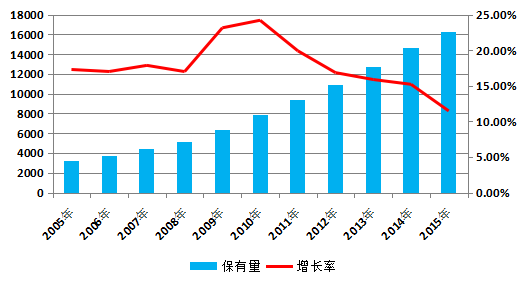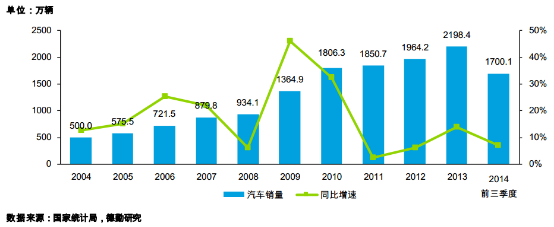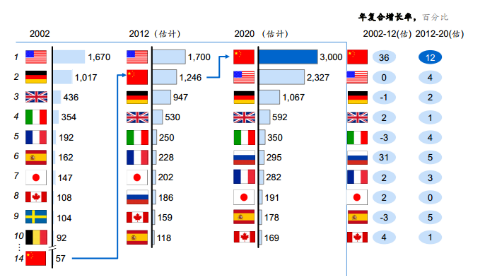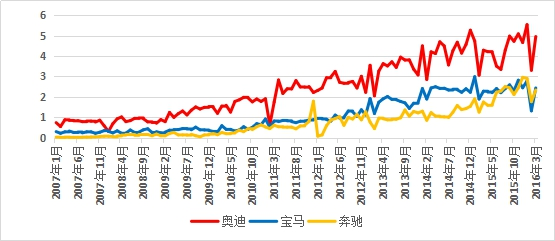## （二）高端车潜在购车人群业务背景分析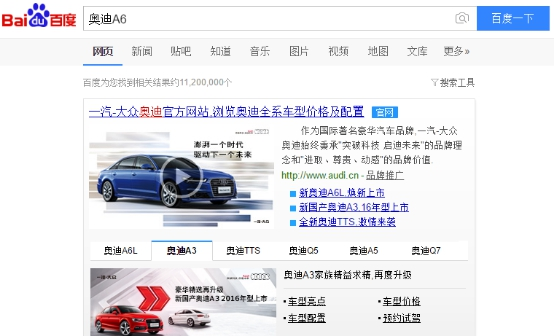# 二、数据描述

## （一）数据概况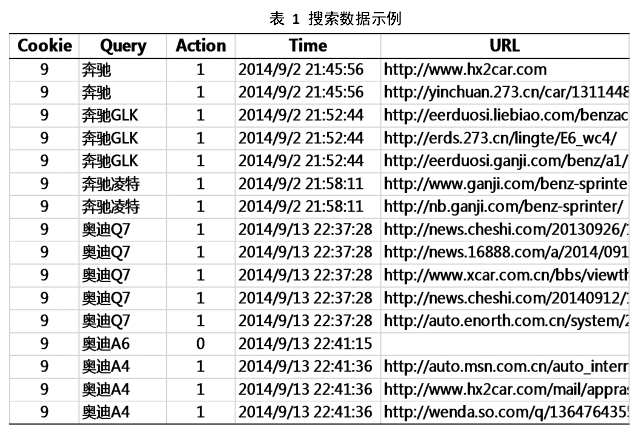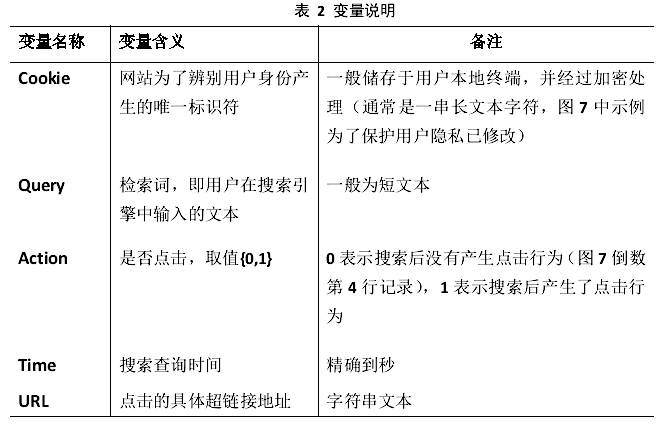## （二）用户画像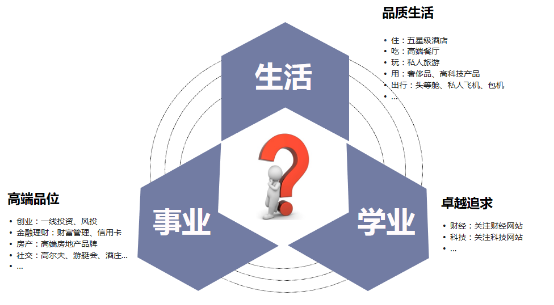## （三）高端车市场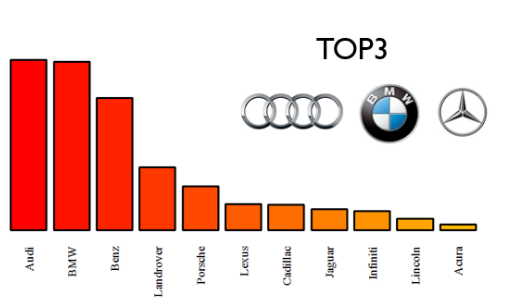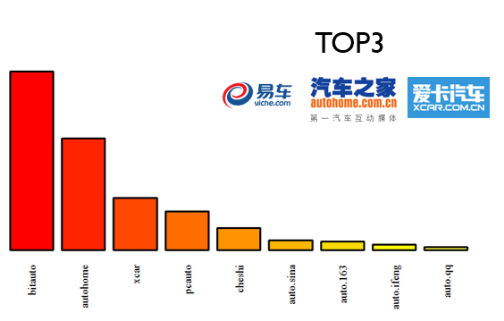$$P_{j_1j_2}=\{\sum_{i=1}^N \sum_{t=1}^{T_i-1}I(Z_{i,t}=j_1)\}^{-1}\{\sum_{i=1}^N\sum_{t=1}^{T_i-1}I(Z_{it}=j_1,Z_{I(t+1)}= j_2)\}$$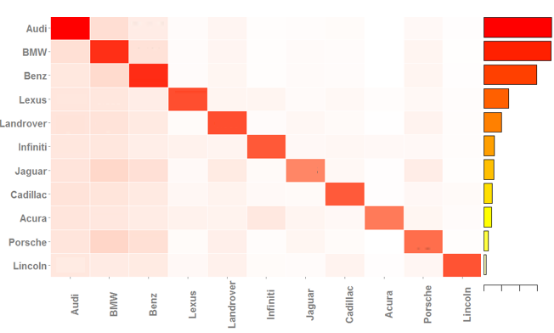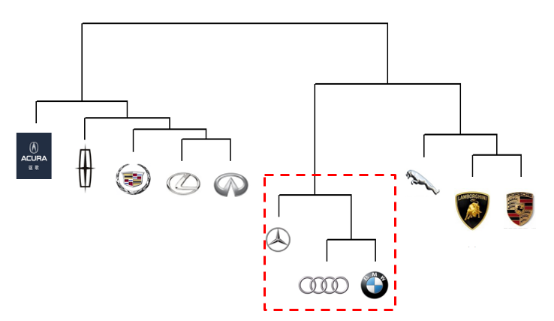# 三、数据建模

## （一）忠诚模型

$$P(Y_{ij}^{(t+1)}=1|Y_{ij}^{(t)}=1)=\frac{exp(\alpha_j+X_i^{(t)’}\beta)}{1+exp(\alpha_j + X_i^{(t)’}\beta)}$$

## （二）影响因素

### （1）搜索品牌数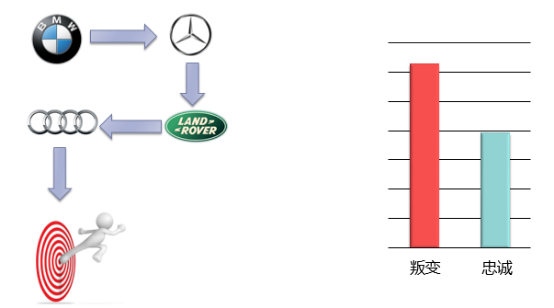### （2）时间间隔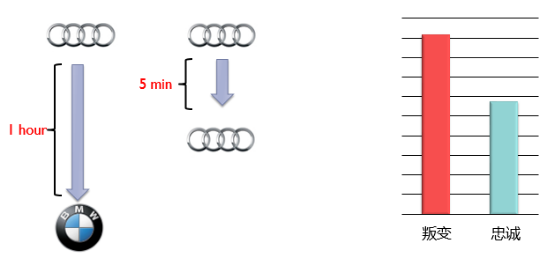### （3）连续搜索数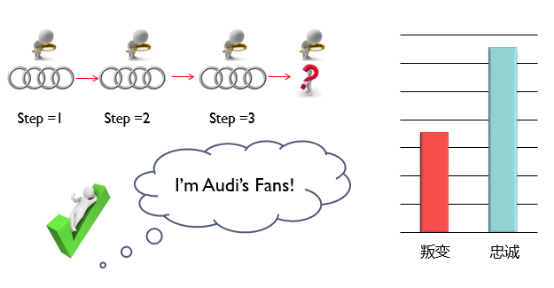### （4）搜索词长度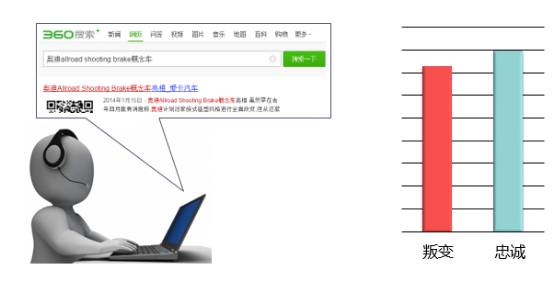### （5）是否搜索配置信息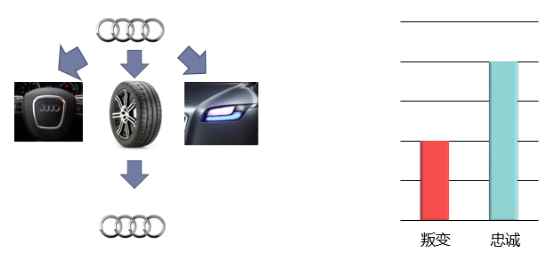### （6）点击数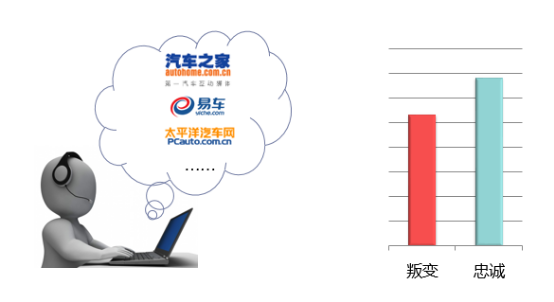### （7）问答百科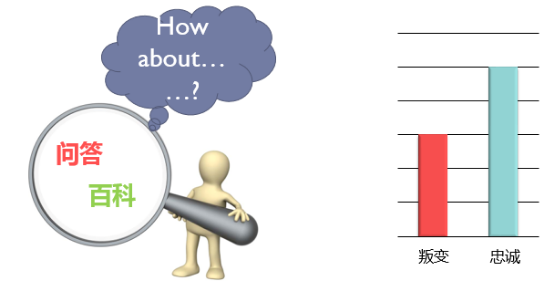## （三）估计结果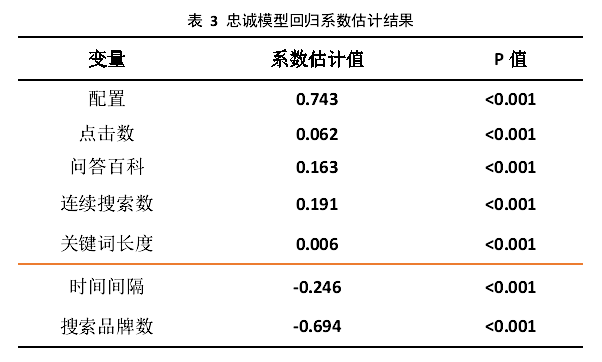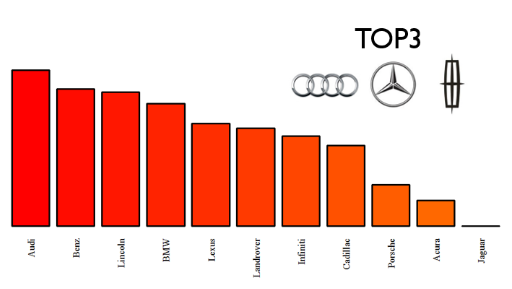## （四）模型评估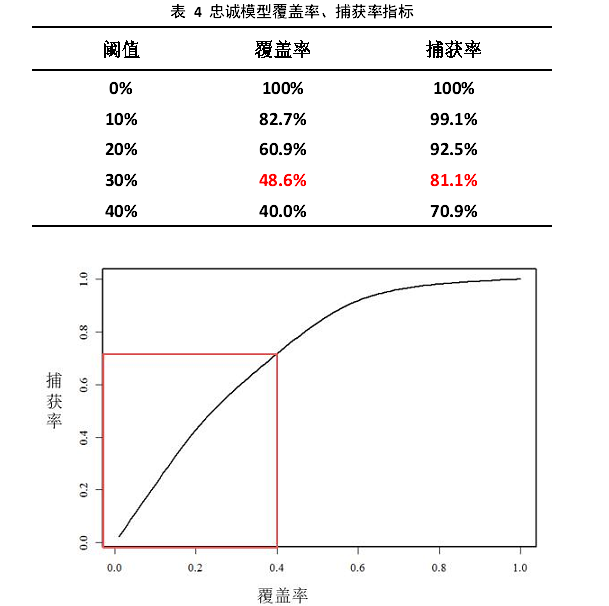# 五、总结与讨论

1. 多数据源信息完善。目前而言，搜索引擎还没有建立完整的用户个人账户体系，但是，这一过程在逐渐完善。如果用户的个人信息资料建立完善，那么就可以更加完整的记录用户的搜索路径，完善其个人特征（例如，年龄、性别等特征）。另一方面，移动互联网的发展使得手机应用逐渐普及，这使得用户的生活轨迹（地理位置、轨迹数据）等可以被完整记录，通过匹配这一数据，可以还原用户的生活特征，并进行更加细节的用户画像。
2. 模型扩展。注意到本文建立的忠诚模型因变量为搜索过程中是否对品牌忠诚，品牌差异性主要体现于模型的截距项参数上。然而，用户对于不同品牌的忠诚度行为模式可能存在更大差异，因此，需要进行更加细节的探索和建模。另外，在已知用户叛变的基础上，未来的研究可对用户对于其他品牌的选择行为进行讨论，从而对竞品投放的广告策略产生更有价值的指导。